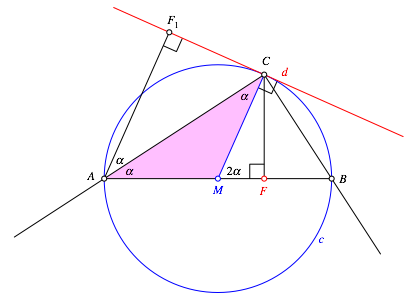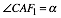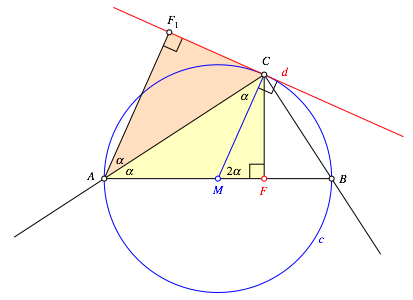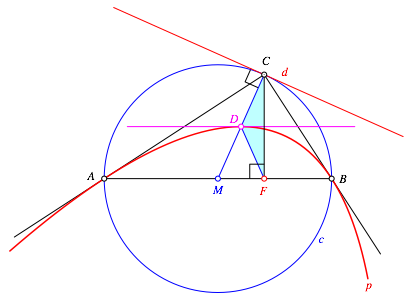Hans Walser, 

Parabola and right triangle

Idea: Abdilkadir Altintas, Afyon, Turkey

We discuss a theorem connecting an arbitrary right triangle to a parabola.

# 2     Situation

Let ABC be a right triangle with right angle at C (Fig. 1). M is the center of the circumcircle c and F the foot of the perpendicular from C to the hypotenuse AB. The line d is the tangent at the circumcircle c in the point C. Of course, the segment MC is orthogonal to the tangent c.Fig. 1: Right triangle

# 3     The theoremFig. 2: Parabola

The parabola p with focus F and directrix d passes through the points A and B and is tangential to the legs CA and CB of the triangle ABC (Fig. 2).

# 4     ProofFig. 3: Angles

The triangle CAM is isosceles. Therefore we have the angle. Now we draw the perpendicular from A to d. The perpendiculars AF1 and MC are parallel. Henceand.Fig. 4: Congruent triangles

Now the triangles CAF and CAF1 are congruent (same angles, side CA in common) (Fig. 4). The triangle CAF1 is just the mirror image of the triangle CAF.

Therefore the distance from A to F is equal to the distance from A to the line d. Hence A is a point of the parabola p. The line AC is angle bisector of the angleand therefore it is tangential to the parabola p.Fig. 5: Argumentation on the other side

An analogue argumentation holds for the point B (Fig. 5).

# 5     More tangents

## 5.1    Parallel to the hypotenuseFig. 6: Tangent parallel to the hypotenuse

The midpoint D of the segment MC is on the parabola p. The tangent in D at the parabola p is parallel to the hypotenuse AB. The proof is given by the isosceles triangle FCD.

## 5.2    Point of intersectionFig. 7: Intersection of three lines

Let S be the point of intersection of the lines d and AB, and E the common point of the parabola p and the line FC. The tangent e at p through E passes also through S. The proof derives from the isosceles triangle FGE.